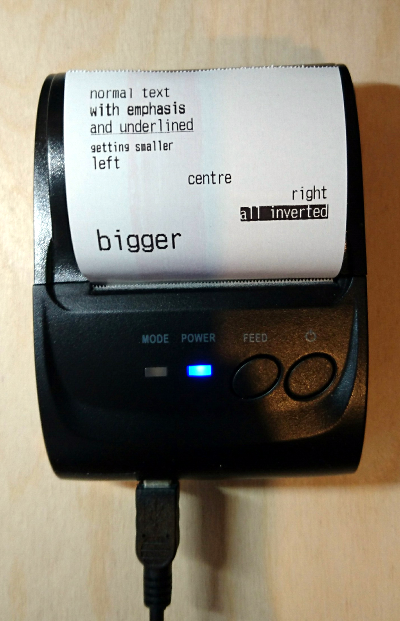keep it simple

# Printing with ESC/POS Printers in Python

##### 2nd December 2016

Small thermal printers commonly used for printing receipts have become more affordable and can be useful for all sorts of projects like interactive exhibits, custom Point Of Sale system or just printing notes.

The ESC/POS specification can be found in this document from EPSON however the functionality actually implemented in each model of printer varies.

This ZJ-5802LD printer from ebay can be connected with USB, serial or Bluetooth and contains a lithium battery allowing printing from mobile phones or tablets in the field.Output from Example

This example implements all the text functionality of the ZJ-5802LD printer and produces the output shown above. We are connecting the printer using the USB port which is presented as `/dev/usb/lp0` in Linux.

In a future post I will cover printing barcodes and images.

``````
#!/usr/bin/env python

NUL = 0x00 # null
LF = 0x0a  # line feed
ESC = 0x1b # escape
GS = 0x1d  # group selector

FONTA = 0x00 # normal
FONTB = 0x01 # small
EMPHASIS = 0x08
DOUBLE_HEIGHT = 0x10
DOUBLE_WIDTH = 0x20
UNDERLINE = 0x80

NORMAL = 0
SMALL = 1

LEFT = 0
CENTRE = 1
RIGHT = 2

def reset(stream):
stream.write(chr(ESC))
stream.write("@")

def mode(stream, value):
stream.write(chr(ESC))
stream.write("!%s" % chr(value))

def emphasis(stream, value):
stream.write(chr(ESC))
stream.write("E%d" % (0, 1)[value])

def underline(stream, value):
stream.write(chr(ESC))
stream.write("-%d" % (0, 1)[value])

def font(stream, value):
assert value >= 0 and value <= 2
stream.write(chr(ESC))
stream.write("M%d" % value)

def justification(stream, value):
assert value >= 0 and value <= 2
stream.write(chr(ESC))
stream.write("a%d" % value)

def inverted(stream, value):
stream.write(chr(GS))
stream.write("B%d" % (0, 1)[value])

def main():
stream = open("/dev/usb/lp0", "wb")

try:
reset(stream)
stream.write("normal text\n")

emphasis(stream, True)
stream.write("with emphasis\n")
emphasis(stream, False)

underline(stream, True)
stream.write("and underlined\n")
underline(stream, False)

font(stream, SMALL)
stream.write("getting smaller\n")
font(stream, NORMAL)

justification(stream, LEFT)
stream.write("left\n")
justification(stream, CENTRE)
stream.write("centre\n")
justification(stream, RIGHT)
stream.write("right\n")

inverted(stream, True)
stream.write("all inverted\n")
inverted(stream, False)

reset(stream)
mode(stream, DOUBLE_WIDTH | DOUBLE_HEIGHT)
stream.write("bigger\n")

stream.flush()

finally:
stream.close()

if __name__ == "__main__":
main()``````